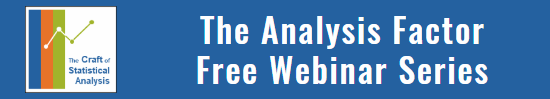# Poisson Regression Analysis for Count Data

by

There are many dependent variables that no matter how many transformations you try, you cannot get to be normally distributed.  The most common culprits are count variables–the variable that measures the count or rate of some event in a sample.  Some examples I’ve seen from a variety of disciplines are:

Number of eggs in a clutch that hatch
Number of domestic violence incidents in a month
Number of times juveniles needed to be restrained during tenure at a correctional facility
Number of infected plants per transect

A common quality of these variables is that 0 is the mode–the most common value.  1 is the next most common, 2 the next, and so on.  In variables with low expected counts (number of cars in a household, number of degrees earned), this is often more pronounced.  No monotonic transformation (log, square root, etc.) can ever move the mode from the end of the distribution to the middle as a normal distribution requires.

But a least-squares normal model doesn’t work for a few more reasons.

First, count variables can’t be below 0.  They just don’t make sense.  But a normal model has no bounds–any value is possible, so a normal model can produce negative predicted values.

Second, with these variables, variance is often not constant–a basic assumption of ordinary least squares regression.  Instead, it goes up with the value of Y.

Another common approach is to categorize the data into two categories–one of the eggs hatched or none did–or more ordered categories–no eggs hatched, 1-2 eggs hatched, 3 or more eggs hatched–then run a Logistic Regression Model.  This can work, but it throws away real information and often lowers power.

As it happens, Count variables often follow a Poisson distribution, and can therefore be used in a Poisson Regression Model.  Poisson Regression Models are similar to Logistic Regression in many ways–they both use Maximum Likelihood Estimation, they both require a transformation of the dependent variable.  Anyone familiar with Logistic Regression will find the leap to Poisson Regression easy to handle.

There are a few issues to keep in mind, though.

1. The link function (the transformation of Y) is the natural log.  So all parameter estimates are on the log scale and need to be transformed for interpretation.

2. It is often necessary to include an exposure or offset parameter in the model to account for the amount of risk each individual had to the event.  A clutch with more eggs will have more opportunity for chicks to hatch.

3. One assumption of Poisson Models is that the mean and the variance are equal, but this assumption is often violated. This can be dealt with by using a dispersion parameter if the difference is small or a negative binomial regression model if the difference is large.

4. Sometimes there are many, many more zeros than even a Poisson Model would indicate.  This generally means there are two processes going on–there is some threshold that needs to be crossed before an event can occur.  A Zero Inflated Poisson Model is a mixture model that simultaneously estimates the probability of crossing the threshold, and once crossed, how many events occur.Poisson and Negative Binomial Regression for Count Data
Learn when you need to use Poisson or Negative Binomial Regression in your analysis, how to interpret the results, and how they differ from similar models.

### Related PostsFrancois BOCQUIER

Laura

Thank you so much for your tutorials, I cannot reach the above link, could you send it ?
Best regards,
FrancoisKarenLaura

https://www.theanalysisfactor.com/poisson-regression-analysis-for-count-data/

“This page contains a link that is broken. By search Poisson on the Institute the topic doesn’t even appear. Can you help me locate the webinar?
If you’d like to learn more about the different models available for Count data, you can download a free recording of the webinar: Poisson and Negative Binomial Regression for Count Data. It’s free.”Karen

Hey Laura,

You should be able to download the webinar here. If you still have trouble, just shoot us an email at support@analysisfactor.com and we’ll help you out.